## 3.3.2 Electron Transport

Simulations with two different setups are conducted: one with a bandgap of 1.89 eV (effective mass 0.11m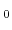in the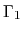valley ), and one with a bandgap of 0.69 eV (effective mass of 0.04m), as summarized in Table 3.4. Results for electron mobility as a function of lattice temperature, free carrier concentration, and electric field are obtained.

As a particular example, Fig. 3.8 shows the low-field electron mobility in hexagonal InN as a function of free carrier concentration. Results from other groups ,, and various experiments ,,,  are also included. Assessing the classical band structure model (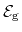=1.89 eV), we achieve an electron mobility of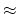4000 cm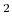/Vs, which is in good agreement with the theoretical results of other groups using a similar setup . Considering the newly calculated band structure model (=0.69 eV), a maximum mobility of10000 cm/Vs is achieved.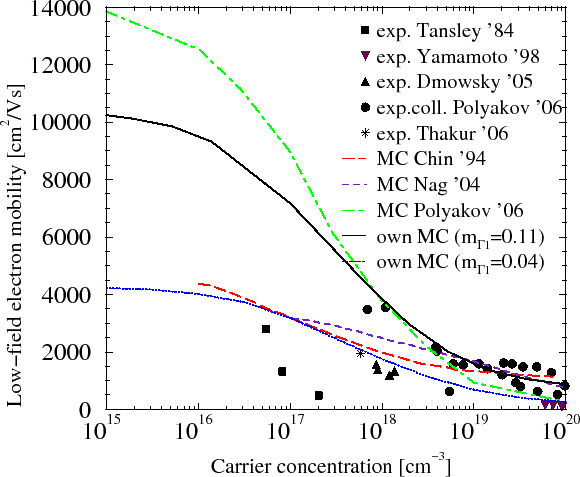The corresponding scattering rates are illustrated in Fig. 3.9. The increased mobility can be explained with the lower effective electron mass. Polyakov et al.  calculated a theoretical limit as high as 14000 cm/Vs, however their simulation does not account for piezoelectric scattering which is the dominant mobility limitation factor at low concentrations (see Fig. 3.9).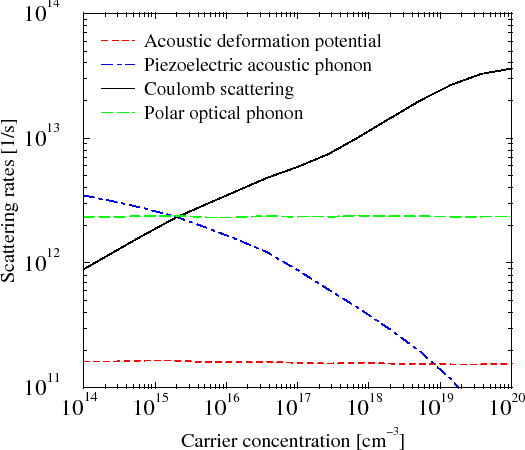Fig. 3.10 shows the mobility as a function of concentration for various values of the polar-optical phonon scattering coefficient and the high-frequency dielectric constant. Choosing the lower value results in a much higher maximum mobility, while the dependence on the scattering coefficient is lower.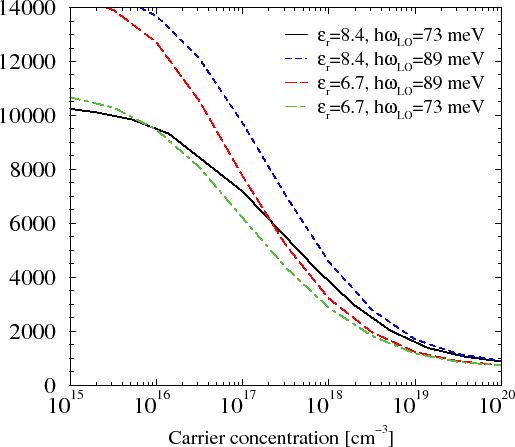Fig. 3.11 shows the electron drift velocity versus electric field at 10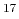cm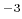carrier concentration. Our MC simulation results differ compared to simulation data from other groups ,,, either due to piezoelectric scattering at lower fields or, at high fields, due to the choice of parameters for the permittivity and polar optical phonon energy (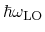).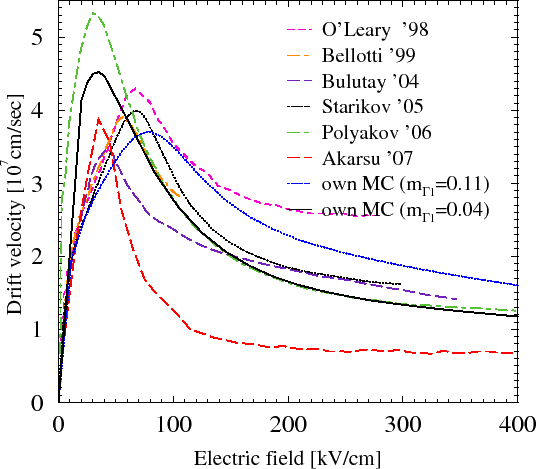Fig. 3.12 shows our simulation results obtained with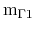=0.04mand with different values of the permittivity and phonon energy. The values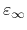=6.7 and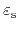=11.0 proposed in  lead to lower electron velocities. Fig. 3.13 confirms that first the polar optical phonon scattering and then the acoustic deformation potential and inter-valley phonon scattering rates increase with higher electric field, and are therefore decisive for the NDM effects.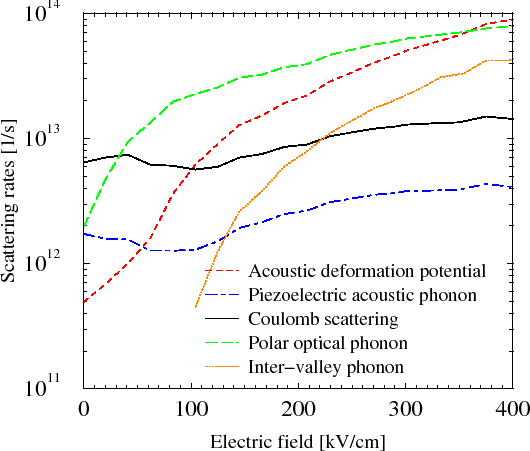S. Vitanov: Simulation of High Electron Mobility Transistors